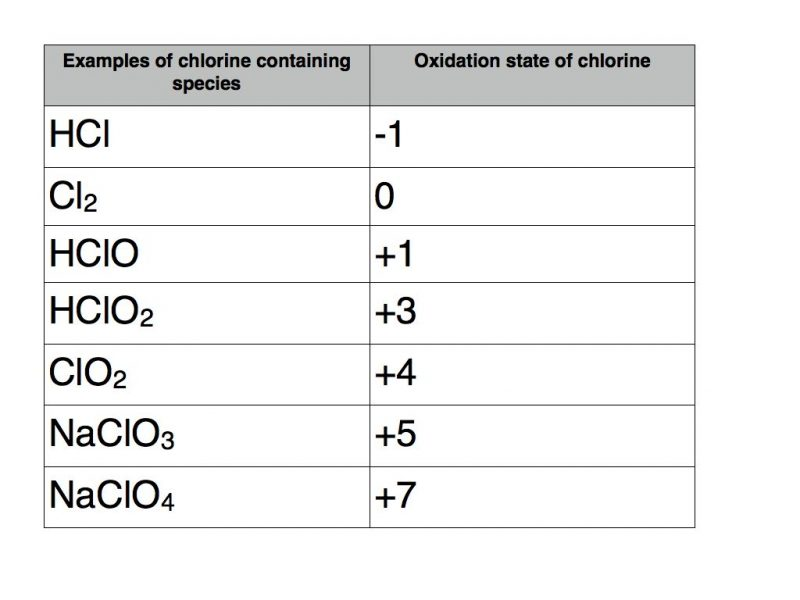# Oxidation state examples

In my last blog post I introduced the concept of oxidation state.  In this blog post you will find a wide variety of worked examples.

The following set of rules should be applied.## Ionic compounds

### Metals in group 1, group 2 and aluminium

The metal will form the positive ion corresponding to the group number as this is the number of electrons in the outer electron shell.  The non metal forms a negative ion with charge equal to the number of electrons required to fill its outer electron shell.

Take magnesium sulphide (MgS) as an example.  Magnesium is in group 2 so it will have an oxidation state of +2.  The compound has no overall charge so the oxidation state of sulphur must be -2.

However in magnesium sulphate (MgSO4) because oxygen must take an oxidation state of -2, sulphur must have a positive number.  In this case sulphur has the oxidation state +6 in order to balance the total oxidation number of -8 from four oxygen atoms.  Magnesium is, of course, still +2.  The full name of this compound is magnesium sulphate (VI).

### Compounds containing a transition metal

Transition metals are renowned for their ability to take a variety of oxidation states.  A classic example is the element manganese.

The table below contains a number of examples of manganese containing compounds with oxidation states varying from +2 to +7.The usual rules apply as far as the other elements are concerned.  Therefore potassium takes oxidation state +1, fluorine -1, oxygen -2 and hydrogen +1.

The example in square brackets is known as a transition metal complex.  It contains six water molecules with coordinate bonds to manganese.  As each water molecule has a total oxidation number of zero they do not contribute to the +2 total oxidation number of the complex which must therefore be solely attributable to manganese.

### Oxidation numbers of nitrogen

Nitrogen can take a variety of oxidation numbers depending upon whether it is bonded to elements that are more or less electronegative than itself.  The following table contains a number of examples.Nitrogen can take any oxidation state between -3 and +5.

A useful rule of thumb for p block elements that exhibit variable oxidation states is that the range of possible oxidation states is between the group number and the group number minus eight.

### Oxidation numbers of chlorine

Applying this rule of thumb to chlorine would indicate that it can take any oxidation state between -1 and +7.  But don’t forget that in a compound it will be -1 unless it is bonded to oxygen of fluorine.  Here are some examples.